var adopen = true; MGR.domReady=function(){ bannerEvt(); resizeEvt(); \$(".manage").mouseenter(function(){ \$(".beta_download").slideDown(200); }).mouseleave(function(){ \$(".beta_download").slideUp(200); }) \$(".sfea").mouseenter(function(){ var h = \$(this).height()*10/178; \$(this).children(".imgcut").children("a").children("img").animate({"top":"-"+h+"px"},200); }).mouseleave(function(){ \$(this).children(".imgcut").children("a").children("img").animate({"top":"0"},200); }) \$(window).resize(); //setTimeout('ad_up()',1000); } function bannerEvt(){ var len = \$(".banner li").length; var index = 0; var isrun = true; \$(".banner li:first").show().css("z-index",1); \$(".b_dot a").mouseenter(function(){ if(index!=\$(".b_dot a").index(this)){ index = \$(".b_dot a").index(this); showImg(index); } isrun = false; }).mouseleave(function(){ isrun = true; }); \$('.banner').bind("mouseenter",function(){ isrun = false; }); \$('.banner').bind("mouseleave",function(){ isrun = true; }) var showImg=function(i){ \$(".banner li,.b_dot a").removeClass("on"); \$(".banner li").eq(i).addClass("on"); \$(".b_dot a").eq(i).addClass("on"); if (\$.browser.msie && (\$.browser.version == "6.0") && !\$.support.style) { \$(".banner li").hide(); \$(".banner li").eq(i).show(); }else{ \$(".banner li").css("z-index",0).fadeOut(600); \$(".banner li").eq(i).css("z-index",1).fadeIn(600); } } showImg(0); //自动开始 var MyTime = setInterval(function(){ if(isrun){ showImg(index) index++; if(index==len){index=0;} } } , 5000); } function resizeEvt(){ \$(window).resize(function(){ \$(".banner").height(\$(".banner").width()*51/127); \$(".figures li .sfea,.figures li .manage").height(\$(".figures").width()*178/1270); var ss = \$(".figures").width()/1270; \$(".manage p").css("margin-bottom",ss*ss*15); if(adopen){ //\$("#adblock").height(\$("#adpic").height()); \$("#adblock").animate({height:\$("#adpic").height()},10); } }); } function ad_up(){ \$("#adblock").animate({height:"63px"},500); \$("#ad_small").animate({bottom:0},500); adopen=false; } function ad_close(){ \$("#ad_wrap").hide(); adopen=true; }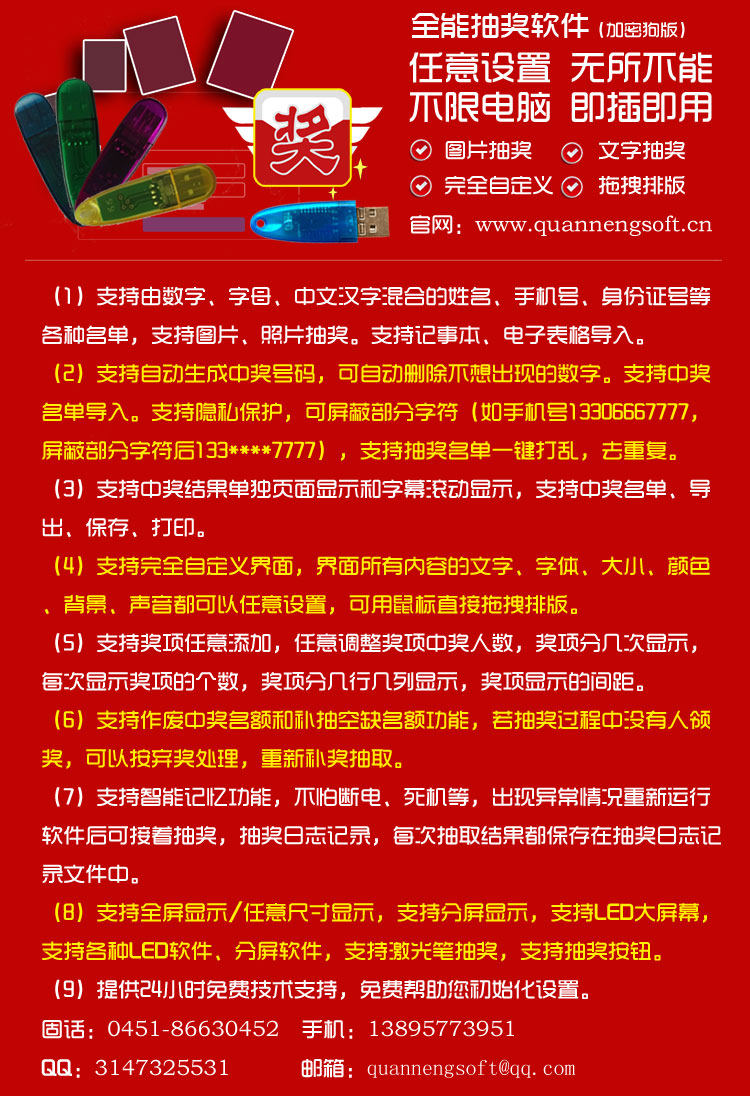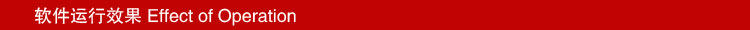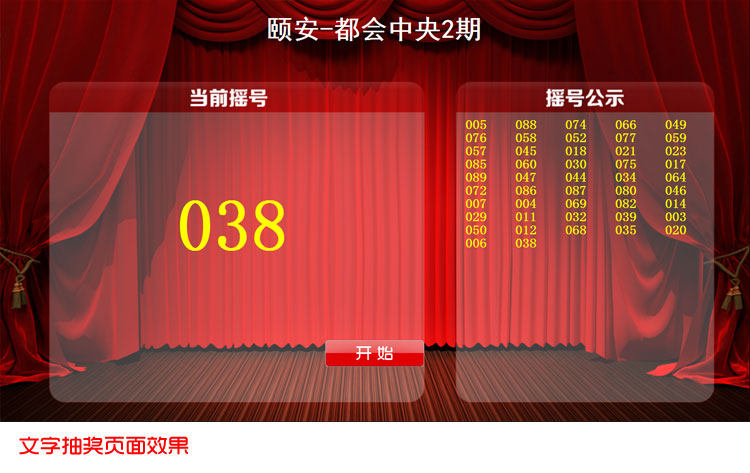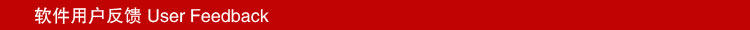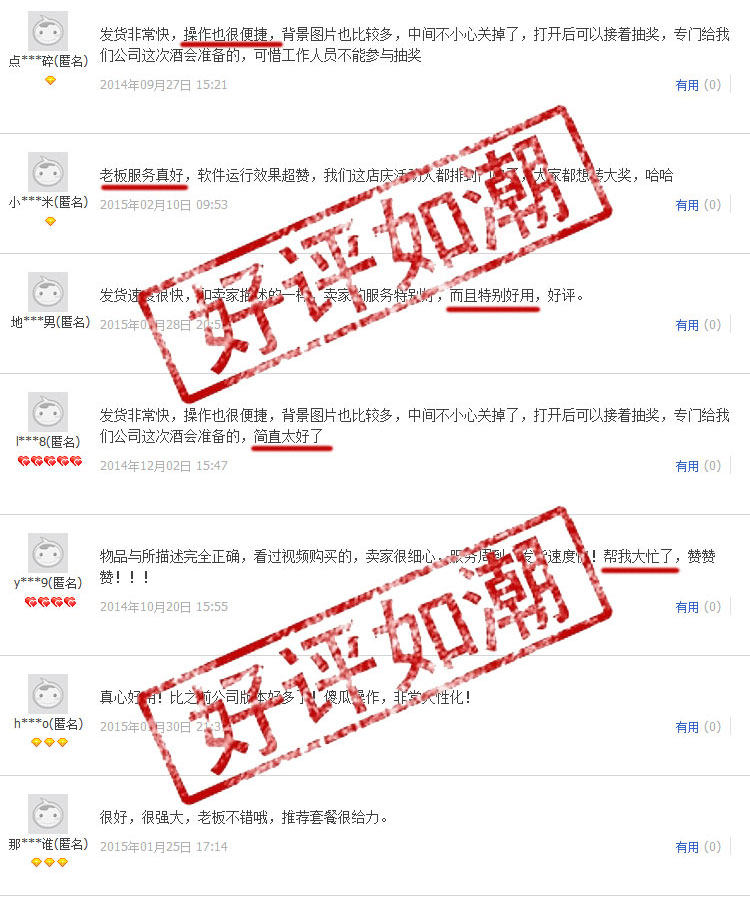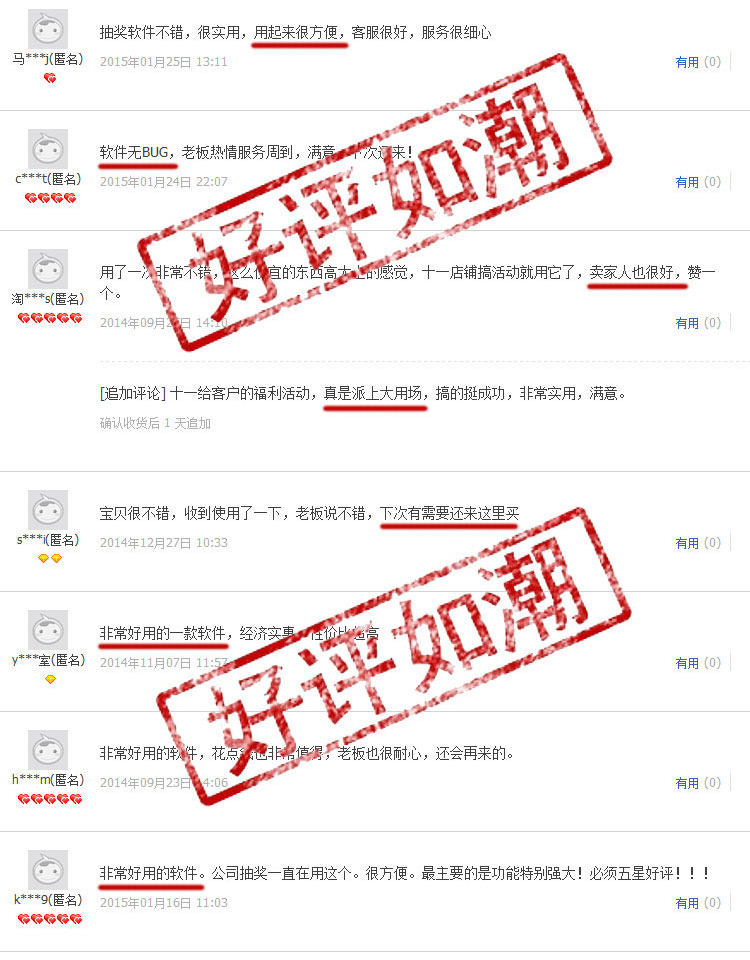xp系统补丁下载(如xp系统无法运行请下载补丁文件)：https://pan.baidu.com/s/1_o11v-H3ok0pZGGXTstBrA

-------------------------------------------------------------------------------------

-------------------------------------------------------------------------------------